# How to Export Multiple Dataframes to Different Excel Worksheets Using R?

Let us look at how to export multiple dataframes to different Excel Worksheets using R language.

xlsx Module:

The xlsx module will be used for this purpose. The xlsx library contains R functions for reading/writing/formatting Excel files and formats. Excel files can be controlled programmatically using the xlsx package. The sheet’s components, such as rows, columns, and cells, can be modified, as well as the sheet fonts, colors, and texts can be formatted.

The file’s contents can be read into a data frame, which is then appended to the sheet. We can install the package into the working space as follows:

Installation:

`install.packages ("xlsx")`

Output:

```> install.packages ("xlsx")
Installing package into ‘C:/Users/cirus/AppData/Local/R/win-library/4.2’
(as ‘lib’ is unspecified)
--- Please select a CRAN mirror for use in this session ---
also installing the dependencies ‘rJava’, ‘xlsxjars’

trying URL 'https://cloud.r-project.org/bin/windows/contrib/4.2/rJava_1.0-6.zip'
Content type 'application/zip' length 1245703 bytes (1.2 MB)

trying URL 'https://cloud.r-project.org/bin/windows/contrib/4.2/xlsxjars_0.6.1.zip'
Content type 'application/zip' length 9485708 bytes (9.0 MB)

trying URL 'https://cloud.r-project.org/bin/windows/contrib/4.2/xlsx_0.6.5.zip'
Content type 'application/zip' length 374759 bytes (365 KB)

package ‘rJava’ successfully unpacked and MD5 sums checked
package ‘xlsxjars’ successfully unpacked and MD5 sums checked
package ‘xlsx’ successfully unpacked and MD5 sums checked

## Exporting Multiple Dataframes to Different Excel Worksheets Using R

Exporting multiple dataframes to different Excel Worksheets can be done using the below methods.

### Method #1: Using R write.xlsx() Method

To write a data frame into an Excel workbook, we use R’s write.xlsx() method. Here, the R object specified as the first argument method is not a dataframe, it can be converted to one.

Syntax:

```write.xlsx(df, file, sheetName = "Sheet1", col.names = TRUE, row.names = TRUE,
append = FALSE, showNA = TRUE, password = NULL )```

Parameters

df: It is the data.frame that will be written in the workbook.

file: It is the path of the output file.

sheetName: It is the name given to the sheet. The default name is “Sheet1”.

col.names: It specifies if the column names of the dataframe(df) must be written to the file.

row.names: It specifies if the row names of the dataframe(df) must be written to the file.

append: It is a logical value that indicates whether or not dataframe(df) should be appended to an existing file.

password: It is a password that is given in a string format.

Example

Approach:

• Import the xlsx module using the library keyword.
• Create a first data frame with some random values using the data. frame and store it in a variable.
• Print the above created first data frame.
• Create a second data frame with some random values using the data. frame and store it in another variable
• Print the above created second data frame
• Give the path of the output file where the result is to be stored and store it in another variable
• Write the content of the first dataframe into the output xlsx file using write.xlsx()function.
• Write the content of the second dataframe(in another sheet) into the output xlsx file using write.xlsx() function.
• The Exit of the Program.

Below is the implementation:

```# Import the xlsx module using the library keyword
library("xlsx")

# Create a first data frame with some random values using the data.frame and store it in a variable
datafrme_1 <- data.frame(col1 = c(1:3),
col2 = letters[1:3],
col3 = TRUE)
# Print the above created first dataframe
print ("The above created first dataframe is:")
print (datafrme_1)

# Create second data frame with some random values using the data.frame and store it in another variable
datafrme_2 <- data.frame(c1 = FALSE,
c2 = rep(1,5),
c3 = seq(0.1,length.out = 5,
by = 0.1))
# Print the above created second dataframe
print("The above created second dataframe is:")
print (datafrme_2)

# Give the path of the output file where the result to be stored and store it in another variable

# Write the content of the first dataframe into the output xlsx file using write.xlsx()
# function
write.xlsx(datafrme_1, file = output_file, sheetName = "sheet1",
row.names = FALSE)

# Write the content of the second dataframe(in another sheet) into the output xlsx file
# using write.xlsx() function
write.xlsx(datafrme_2, file= output_file, sheetName = "sheet2",
append = TRUE, row.names = FALSE)
```

Output:

``` "The above created first dataframe is:"

col1 col2 col3
1 1 a TRUE
2 2 b TRUE
3 3 c TRUE

 "The above created second dataframe is:"

c1 c2 c3
1 FALSE 1 0.1
2 FALSE 1 0.2
3 FALSE 1 0.3
4 FALSE 1 0.4
5 FALSE 1 0.5```

Output Excel File: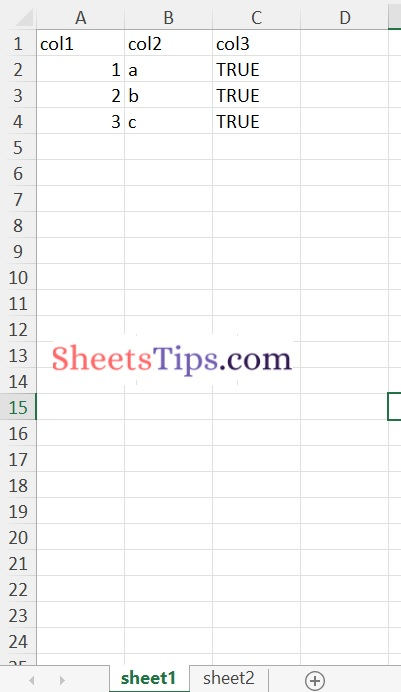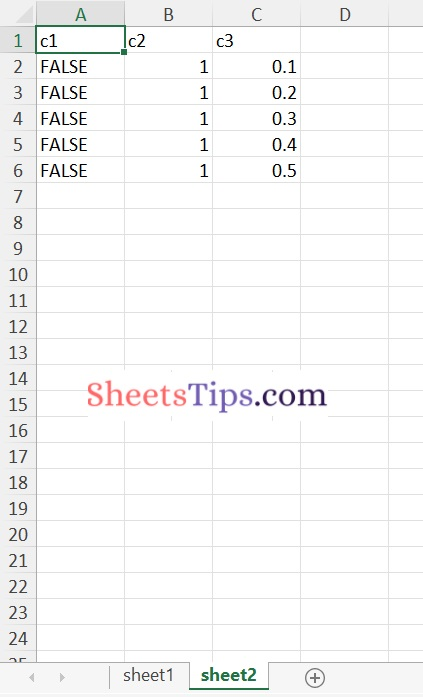### Method #2: Using R createWorkbook() Method

1)createWorkbook() method:

In R, the createWorkbook() method is used to generate/create an empty workbook object. It returns a java object with a reference to an empty object.

`createWorkbook(type = "xlsx")`

2)createSheet() Function:

In R, the createSheet() method returns the created sheet object. The workbook object can be used to call createSheet().

`createSheet(wb, sheetName)`

In R, we use the addDataFrame() method to add a data frame to a sheet, which allows for different column styles. This solution is preferable to the previous one since it allows you to customize both rows and columns.

Syntax:

```addDataFrame( df , sheetName, col.names = TRUE, row.names = TRUE, startRow = 1,
startColumn = 1)```

Parameters

df: It is the data.frame that will be written in the workbook.

sheetName: It is the name given to the sheet. The default name is “Sheet1”.

col.names: It specifies if the column names of the dataframe(df) must be written to the file.

row.names: It specifies if the row names of the dataframe(df) must be written to the file.

startRow: It indicates the starting row.

startColumn: It indicates the starting column.

4)saveWorkbook() Function:

The workbook can be saved with the saveWorkbook() method.

Syntax:

`saveWorkbook(workbook, file, password = NULL)`

Example

Approach:

• Import the xlsx module using the library keyword.
• Create a first data frame with some random values using the data. frame and store it in a variable.
• Print the above created first data frame.
• Create second data frame with some random values using the data.frame and store it in another variable.
• Print the above created second dataframe.
• Similarly Create the third dataframe.
• Print the above created third dataframe.
• Give the path of the output file where the result is to be stored and store it in another variable.
• Create a workbook object using the createWorkbook() function.
• Create a new sheet using the createSheet() function by passing the above workbook object, sheet name as arguments to it.
• Add the first dataframe to the above created first sheet using the addDataFrame() function.
• Create a new secondsheet using the createSheet() function by passing the above workbook object, sheet name as arguments to it.
• Add the second dataframe to the above created second sheet using the addDataFrame() function
• Similarly do it for the third dataframe.
• Save the above workbook object using the saveWorkbook() function by passing the workbook object, output file path as arguments to it.
• The Exit of the Program.

Below is the implementation:

```# Importing xlsx module using the library keyword
library("xlsx")

# Create a first data frame with some random values using the data.frame and store it in a variable
datafrme_1 <- data.frame(col1 = c(1:3),
col2 = letters[1:3],
col3 = TRUE)
# Print the above created first dataframe
print ("The above created first dataframe is:")
print (datafrme_1)

# Create second data frame using the data.frame and store it in another variable
datafrme_2 <- data.frame(c1 = FALSE,
c2 = rep(1,5),
c3 = seq(0.1,length.out = 5,
by = 0.1))
# Print the above created second dataframe
print("The above created second dataframe is:")
print (datafrme_2)

# Similarly Create the third dataframe
datafrme_3 <- data.frame(c1 = FALSE,
c2 = rep(1,5),
c3 = seq(0.1,length.out = 5,
by = 0.1))
# Print the above created third dataframe
print("The above created third dataframe is:")
print (datafrme_3)

# Give the path of the output file and store it in another variable

# Create a workbook object using the  createWorkbook() function
workbookObj = createWorkbook()
# Create a new sheet using the createSheet() function by passing the above workbook
# object, sheet name as arguments to it
newWorkSheet_1 = createSheet(workbookObj, "FirstSheet")

# Add the first dataframe to the above created first sheet using the addDataFrame() function
row.names = FALSE)

# Create a new secondsheet using the createSheet() function by passing the above workbook
# object, sheet name as arguments to it
newWorkSheet_2 = createSheet(workbookObj, "SecondSheet")

# Add the second dataframe to the above created second sheet using the addDataFrame() function
addDataFrame(datafrme_2, sheet = newWorkSheet_2, startColumn = 1,
row.names = FALSE)

# Similarly do it for the third dataframe
newWorkSheet_3 = createSheet(workbookObj, "ThirdSheet")

addDataFrame(datafrme_3, sheet = newWorkSheet_3, startColumn = 1,
row.names = FALSE)

# Save the above workbook object using the saveWorkbook() function
# by passing the workbook object, output file path as arguments to it
saveWorkbook(workbookObj, output_file)```

Output:

``` "The above created first dataframe is:"

col1 col2 col3
1 1 a TRUE
2 2 b TRUE
3 3 c TRUE

 "The above created second dataframe is:"

c1 c2 c3
1 FALSE 1 0.1
2 FALSE 1 0.2
3 FALSE 1 0.3
4 FALSE 1 0.4
5 FALSE 1 0.5
 "The above created third dataframe is:"
c1 c2 c3
1 FALSE 1 0.1
2 FALSE 1 0.2
3 FALSE 1 0.3
4 FALSE 1 0.4
5 FALSE 1 0.5```

Output Excel File: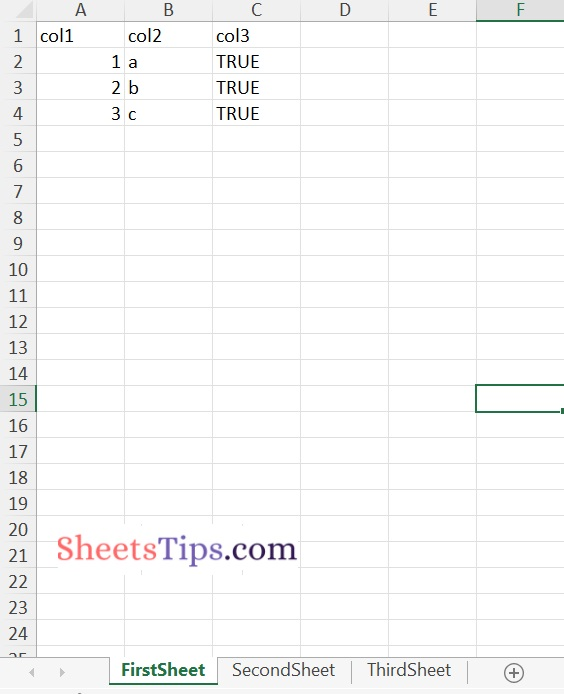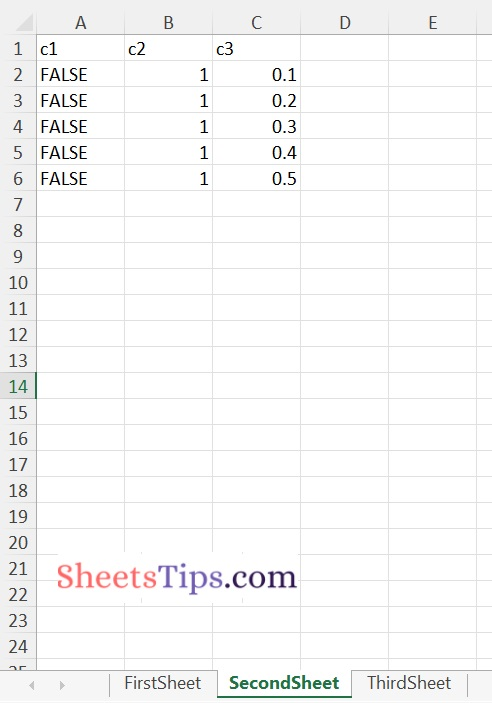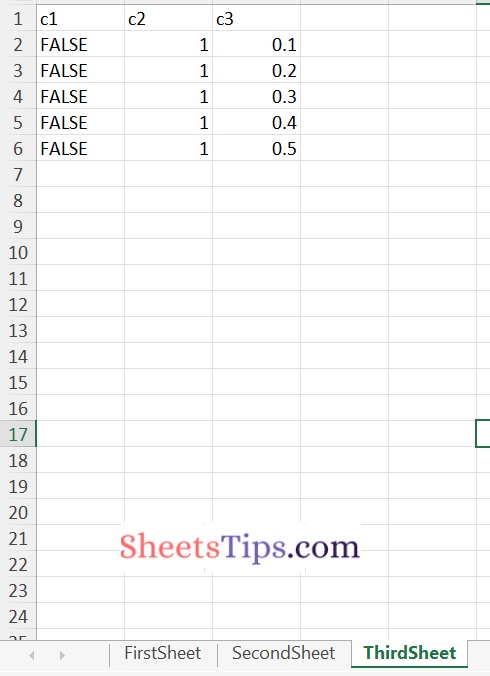### Merging Multiple DataFrames and storing them in an excel file:

Approach:

• Import the xlsx module using the library keyword.
• Create a first data frame with some random values using the data. frame and store it in a variable.
• Print the above created first data frame.
• Create second data frame with some random values using the data.frame and store it in another variable.
• Print the above created second dataframe.
• Similarly Create the third dataframe.
• Print the above created third dataframe.
• Merge the above two created data frames with the common column c2 using merge() function and store it in a new #dataframe say data frame 4.
• Print the merged dataframe
• Give the path of the output file where the result is to be stored and store it in another variable.
• Create a workbook object using the createWorkbook() function.
• Create a new sheet using the createSheet() function by passing the above workbook object, sheet name as arguments to it.
• Add the first dataframe to the above created first sheet using the addDataFrame() function.
• Create a new secondsheet using the createSheet() function by passing the above workbook object, sheet name as arguments to it here this second sheet stores all the merged values of the data frame 2 and data frame 3.
• Add the dataframe 4 to the above created second sheet using the addDataFrame() function
• Save the above workbook object using the saveWorkbook() function by passing the workbook object, output file path as arguments to it.
• The Exit of the Program.

Below is the Implementation:

```# Importing xlsx module using the library keyword
library("xlsx")

# Create a first data frame with some random values using the data.frame and store it in a variable
datafrme_1 <- data.frame(col1 = c(1:3),
col2 = letters[1:3],
col3 = TRUE)
# Print the above created first dataframe
print ("The above created first dataframe is:")
print (datafrme_1)

# Create second data frame using the data.frame and store it in another variable
datafrme_2 <- data.frame(c1 = FALSE,
c2 = rep(1,5),
c3 = seq(0.1,length.out = 5,
by = 0.1))
# Print the above created second dataframe
print("The above created second dataframe is:")
print (datafrme_2)

# Similarly Create the third dataframe
datafrme_3 <- data.frame(c1 = FALSE,
c2 = rep(1,5),
c3 = seq(0.1,length.out = 5,
by = 0.1))
# Print the above created third dataframe
print("The above created third dataframe is:")
print (datafrme_3)

# Merge the above two created data frames with the common column c2 using merge() function and store it in a new #dataframe say dataframe 4
datafrme_4<-merge(datafrme_2, datafrme_3, by="c1")

#Print the merged dataframe
print("The merged data frame of second and third dataframe is :")
print(datafrme_4)

# Give the path of the output file and store it in another variable

# Create a workbook object using the  createWorkbook() function
workbookObj = createWorkbook()
# Create a new sheet using the createSheet() function by passing the above workbook
# object, sheet name as arguments to it
newWorkSheet_1 = createSheet(workbookObj, "FirstSheet")

# Add the first dataframe to the above created first sheet using the addDataFrame() function
row.names = FALSE)

# Create a new secondsheet using the createSheet() function by passing the above workbook
# object, sheet name as arguments to it
newWorkSheet_2 = createSheet(workbookObj, "SecondSheet")

# Add the second dataframe to the above created second sheet using the addDataFrame() function
addDataFrame(datafrme_4, sheet = newWorkSheet_2, startColumn = 1,
row.names = FALSE)

# Save the above workbook object using the saveWorkbook() function
# by passing the workbook object, output file path as arguments to it
saveWorkbook(workbookObj, output_file)```

Output:

``` "The above created first dataframe is:"

col1 col2 col3
1 1 a TRUE
2 2 b TRUE
3 3 c TRUE

 "The above created second dataframe is:"

c1 c2 c3
1 FALSE 1 0.1
2 FALSE 1 0.2
3 FALSE 1 0.3
4 FALSE 1 0.4
5 FALSE 1 0.5
  "The merged data frame of second and third dataframe is :"
c1 c2.x c3.x c2.y c3.y
1 FALSE 1 0.1 1 0.1
2 FALSE 1 0.1 1 0.2
3 FALSE 1 0.1 1 0.3
4 FALSE 1 0.1 1 0.4
5 FALSE 1 0.1 1 0.5
6 FALSE 1 0.2 1 0.1
7 FALSE 1 0.2 1 0.2
8 FALSE 1 0.2 1 0.3
9 FALSE 1 0.2 1 0.4
10 FALSE 1 0.2 1 0.5
11 FALSE 1 0.3 1 0.1
12 FALSE 1 0.3 1 0.2
13 FALSE 1 0.3 1 0.3
14 FALSE 1 0.3 1 0.4
15 FALSE 1 0.3 1 0.5
16 FALSE 1 0.4 1 0.1
17 FALSE 1 0.4 1 0.2
18 FALSE 1 0.4 1 0.3
19 FALSE 1 0.4 1 0.4
20 FALSE 1 0.4 1 0.5
21 FALSE 1 0.5 1 0.1
22 FALSE 1 0.5 1 0.2
23 FALSE 1 0.5 1 0.3
24 FALSE 1 0.5 1 0.4
25 FALSE 1 0.5 1 0.5```